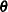The MI Procedure
 Parameter Simulation versus Multiple Imputation

As an alternative to multiple imputation, parameter simulation can also be used to analyze the data for many incomplete-data problems. Although the MI procedure does not offer parameter simulation, the trade-offs between the two methods (Schafer 1997, pp. 89–90, 135–136) are examined in this section.

The parameter simulation method simulates random values of parameters from the observed-data posterior distribution and makes simple inferences about these parameters (Schafer 1997, p. 89). When a set of well-defined population parametersare of interest, parameter simulation can be used to directly examine and summarize simulated values of. This usually requires a large number of iterations, and involves calculating appropriate summaries of the resulting dependent sample of the iterates of the. If only a small set of parameters are involved, parameter simulation is suitable (Schafer 1997).

Multiple imputation requires only a small number of imputations. Generating and storing a few imputations can be more efficient than generating and storing a large number of iterations for parameter simulation.

When fractions of missing information are low, methods that average over simulated values of the missing data, as in multiple imputation, can be much more efficient than methods that average over simulated values ofas in parameter simulation (Schafer 1997).Previous Page | Next Page | Top of Page